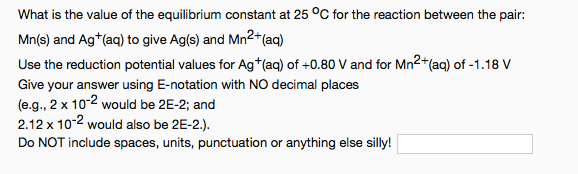# What is the value of the equilibrium constant at 25°C for the reaction between the pair: Mn(s) and Ag+(aq) to give Ag(s) and Mn2+(aq) Use the reduction potential values for Ag+(aq) of +0.80 V and for Mn2+(aq) of -1.18 V. Give your answer using E-notation with NO decimal places (e.g., 2 x 10^-2 would be 2E-2; and 2.12 x 10^-2 would also be 2E-2.).